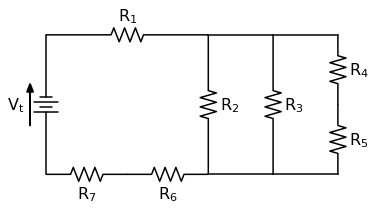## How to add an Inset Curve with Matplotlib and PythonIn this post, you'll see how to add an inset curve to a Matplotlib plot. An inset curve is a small plot laid on top of a main larger plot. The inset curve is smaller than the main plot and typically shows a "zoomed in" region of the main plot …

## Plotting a Stress Strain Curve with Python and MatplotlibIn this post, we'll use data from a tensile test to build a stress strain curve with Python and Matplotlib.

A tensile test is a type of mechanical test performed by engineers used to determine the mechanical properties of a material. Engineering metal alloys such as steel and aluminum alloys …

## Plotting a stress-strain curve with four libraries: matplotlib, pandas, altair and bokehAfter watching a great webinar about plotting with different python libraries, I wanted to see what it was like to make a stress strain curve using four different modules: pandas, matplotlib, altair and bokeh (with holoviews).

## Solving a Circuit Diagram Problem with Python and SchemDraw

In this post we will solve a circuit diagram problem using a Python package called SchemDraw## Diffusion Calculation with Python and Pint

I was working on an engineering problem involving diffusion that involved a couple of different units including joules, grams, kilograms, meters, centimeters, moles, megapascals and weight percent. To make sure that I kept track of all the units and unit conversions throughout the problem, I thought I'd try using pint, a Python package for unit conversions.